# Arduino 直流电机

• DC motor 直流电机
• Servo motor 伺服电机
• Stepper motor 步进电机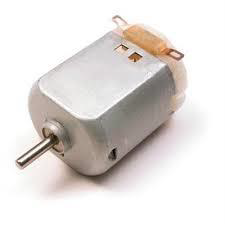• 只让你的电机旋转
• 控制电机速度
• 控制直流电机的旋转方向

### 必需的组件

• 1x Arduino UNO 板
• 1x PN2222 晶体管
• 1x 小型6V直流电机
• 1x 1N4001二极管
• 1x 270Ω电阻

### 程序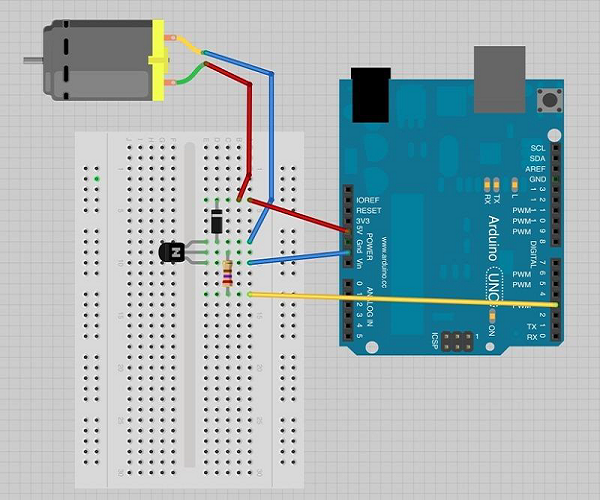### 预防措施

• 首先，确保晶体管以正确的方式连接。如图所示，晶体管的扁平面应该面向Arduino板。

• 其次，根据图像中所示的排列，二极管的条纹端应朝向+5V电源线。

### Arduino旋转控制代码

```int motorPin = 3;

void setup() {

}

void loop() {
digitalWrite(motorPin, HIGH);
}
```

## 电机速度控制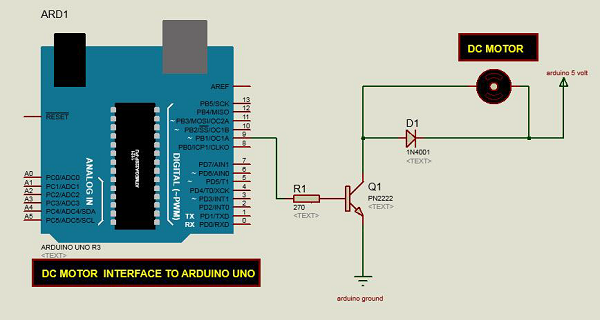### Arduino代码

```int motorPin = 9;

void setup() {
pinMode(motorPin, OUTPUT);
Serial.begin(9600);
while (! Serial);
Serial.println("Speed 0 to 255");
}

void loop() {
if (Serial.available()) {
int speed = Serial.parseInt();
if (speed >= 0 && speed <= 255) {
analogWrite(motorPin, speed);
}
}
}```

### 代码说明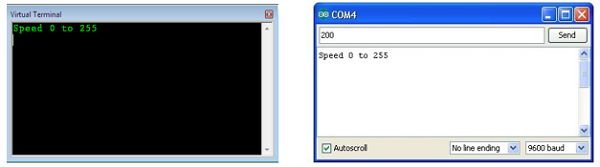## 旋转方向控制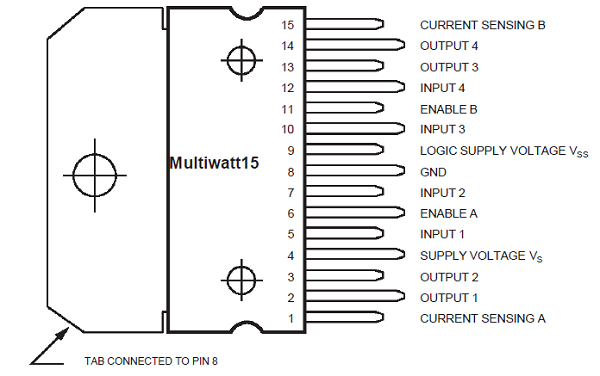### 必需的组件

• 1 × L298桥式IC
• 1 × 直流电机
• 1 × Arduino UNO
• 1 × 面包板
• 10 × 跳线

### 程序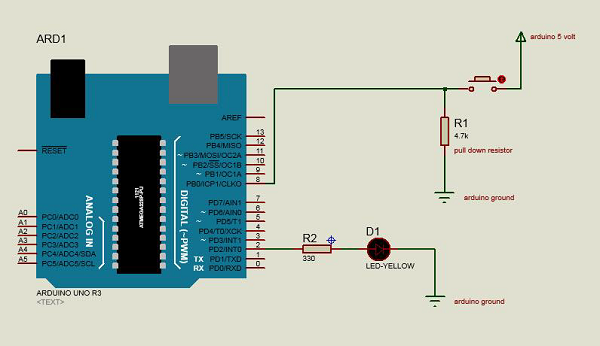IN1IN2电机行为
制动
1 向前
1向后
11制动

IC L298的引脚IN1连接到Arduino的引脚8，而IN2连接到引脚9。Arduino的这两个数字引脚控制电机的方向。IC的EN A引脚连接到Arduino的PWM引脚2。这将控制电机的速度。

### 连接步骤

• 将IC的5V和接地分别连接到Arduino的5V和接地。
• 将电机连接到IC的引脚2和3。
• 将IC的IN1连接到Arduino的引脚8。
• 将IC的IN2连接到Arduino的引脚9。
• 将IC的EN1连接到Arduino的引脚2。
• 将ICD的SENS A引脚接地。
• 使用Arduino USB线连接Arduino，并使用Arduino IDE软件将程序上传到Arduino。
• 使用电源，电池或USB线为Arduino板供电。

### Arduino代码

```const int pwm = 2 ; //initializing pin 2 as pwm
const int in_1 = 8 ;
const int in_2 = 9 ;
//For providing logic to L298 IC to choose the direction of the DC motor

void setup() {
pinMode(pwm,OUTPUT) ; //we have to set PWM pin as output
pinMode(in_1,OUTPUT) ; //Logic pins are also set as output
pinMode(in_2,OUTPUT) ;
}

void loop() {
//For Clock wise motion , in_1 = High , in_2 = Low
digitalWrite(in_1,HIGH) ;
digitalWrite(in_2,LOW) ;
analogWrite(pwm,255) ;
/* setting pwm of the motor to 255 we can change the speed of rotation
by changing pwm input but we are only using arduino so we are using highest
value to driver the motor */
//Clockwise for 3 secs
delay(3000) ;
//For brake
digitalWrite(in_1,HIGH) ;
digitalWrite(in_2,HIGH) ;
delay(1000) ;
//For Anti Clock-wise motion - IN_1 = LOW , IN_2 = HIGH
digitalWrite(in_1,LOW) ;
digitalWrite(in_2,HIGH) ;
delay(3000) ;
//For brake
digitalWrite(in_1,HIGH) ;
digitalWrite(in_2,HIGH) ;
delay(1000) ;
}```

### 结果

App下载